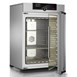Hi, Guest!
• 0

# Extech DT200: Laser Distance Meter

Update Terakhir
:
01 / 12 / 2019
Min. Pembelian
:
1 Unit

### Detail Extech DT200: Laser Distance Meter

Extech DT200: Laser Distance Meter

## Laser measurements up to 115ft (35m)

The DT200 features a laser measurement accurate to 0.08 in. at 32 ft. Measures from 2 in. to 115 ft. (0.05 to 35 m). Historical Storage recalls the previous 10 records (measurements or calculations). Automatically calculates Area and Volume. Indirect measurement using Pythagorean Theorem. Continuous measurement function with Min/Max distance tracking updates every 5 seconds. Addition/Subtraction, Front or rear edge reference. Low battery indicator, Auto power off. Complete with carrying case and 2 AAA batteries.

• Measures from 2" to 115' (0.05 to 35m)
• Laser measurement accurate to 0.08 inches at 32 feet
• Historical Storage recalls the previous 10 records (measurements or calculated results)
• Automatically calculates Area and Volume
• Indirect measurement using Pythagorean theorem
• Continuous measurement function with Min/Max distance tracking updates every 5 seconds
• Addition/Subtraction, Front or rear edge reference
• Low battery indicator, Auto power off
• Complete with carrying case and 2 AAA batteries
Specifications Range
Measurement Range 2" to 115' (0.05 to 35m)
Accuracy (up to 32'/10m) ±0.08" (±2mm)
Resolution 0.001" (0.001m)
Area calculation 999.99 sq. ft. (999.99m2)
Volume calculation 999.99 cu. ft. (999.99m3)
Laser Power Off after 30 seconds
Auto Power Off after 3 minutes
Power Supply 2 AAA Batteries
Dimensions 4.5 x 1.9 x 1.1" (115 x 48 x 28mm)
Weight 5.3oz (150g)### PT ANDALAN BANGUN SEJAHTERA

JL. Sumur Batu No.1 RT.01 - RW.06 Kel.Sumur Batu - Kec.. Kemayoran, Jakarta Pusat
Indonesia 10640
Jakarta Pusat
-

### Detail Extech DT200: Laser Distance Meter

Extech DT200: Laser Distance Meter

## Laser measurements up to 115ft (35m)

The DT200 features a laser measurement accurate to 0.08 in. at 32 ft. Measures from 2 in. to 115 ft. (0.05 to 35 m). Historical Storage recalls the previous 10 records (measurements or calculations). Automatically calculates Area and Volume. Indirect measurement using Pythagorean Theorem. Continuous measurement function with Min/Max distance tracking updates every 5 seconds. Addition/Subtraction, Front or rear edge reference. Low battery indicator, Auto power off. Complete with carrying case and 2 AAA batteries.

• Measures from 2" to 115' (0.05 to 35m)
• Laser measurement accurate to 0.08 inches at 32 feet
• Historical Storage recalls the previous 10 records (measurements or calculated results)
• Automatically calculates Area and Volume
• Indirect measurement using Pythagorean theorem
• Continuous measurement function with Min/Max distance tracking updates every 5 seconds
• Addition/Subtraction, Front or rear edge reference
• Low battery indicator, Auto power off
• Complete with carrying case and 2 AAA batteries
Specifications Range
Measurement Range 2" to 115' (0.05 to 35m)
Accuracy (up to 32'/10m) ±0.08" (±2mm)
Resolution 0.001" (0.001m)